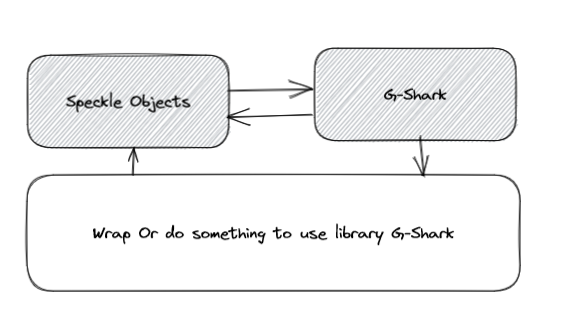# Discuss about Improvement Geometry Objects Library

I have some problem need discuss about Library Speckle Objects:

1. Replace some method static like `CrossProduc`t and `DotProduct` for easy use API :

Current :

``````/// <summary>
/// Computes the vector product (cross product) of two given vectors
/// Cross product = { u2 * v3 - u3 * v2; u3 * v1 - u1 * v3; u1 * v2 - u2 * v1 }.
/// </summary>
/// <param name="u">First vector.</param>
/// <param name="v">Second vector.</param>
/// <returns>Vector result of the cross product.</returns>
public static Vector CrossProduct(Vector u, Vector v)
{
var x = u.y * v.z - u.z * v.y;
var y = u.z * v.x - u.x * v.z;
var z = u.x * v.y - u.y * v.x;

return new Vector(x, y, z);
}
``````

Can replace by :

``````/// <summary>
/// Computes the vector product (cross product) of two given vectors
/// Cross product = { u2 * v3 - u3 * v2; u3 * v1 - u1 * v3; u1 * v2 - u2 * v1 }.
/// </summary>
/// <param name="v">Second vector.</param>
/// <returns>Vector result of the cross product.</returns>
public Vector CrossProduct(Vector v)
{
var x = this.y * v.z - this.z * v.y;
var y = this.z * v.x - this.x * v.z;
var z = this.x * v.y - this.y * v.x;

return new Vector(x, y, z);
}
``````

This will be help all developer easy use API and code look beter , how you think about this ?
At the moment with API :

``````  void DoSomeThing()
{
Point p1 = new Point(0, 0, 0);
Point p2 = new Point(4, 0, 0);
Vector vector = new Vector(p1);
Vector vector2 = new Vector(p2);
var result = Vector.DotProduct(vector,vector2);
}
``````

After change :

``````        void DoSomeThingImprove()
{
Point p1 = new Point(0, 0, 0);
Point p2 = new Point(4, 0, 0);
var result = p1.DotProduct(p2);
}
``````

Note : This just a example for that, many method inside code is same now.

1. Will be add libary G-Shark to use and Upgrade for this, but I see base code current is not easy to change.If don’t have any problem, I will try pull request some hack help this1 Like